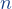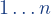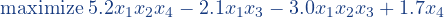# The BINPOLOPT Format

Rules:

• each line starting with a “c” is considered a comment and thus skipped
• the first (non-comment) line contains an integer[meaning:is the number of variables]

every following (non-comment) line (at most 10000 characters) represents a monomial. It contains a sequence of terms separated by an arbitrary number of characters in the set (<space>, <tab>,  <comma>, <semicolon>) the first term is a real (in fixed or floating-point representation) or an integer representing the weight of the monomial in the objective function (note that the objective function is always maximized) the following terms are integers in the range, representing the indices of all the variables appearing in the monomial a line containing only characters in the set (<space>, <tab>, <comma>, <semicolon>) does not provide the definition of any monomial but is accepted

Example:Input file:

c
c Binary Polynomial Optimization Instance
c
4
5.2   1 2 4
-2.1  1 3
-3.0  1 2 3
1.7   4